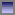USIT/CrePS Presentation: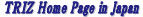Tutorial: USIT (Unified Structured Inventive Thinking) in the Six-Box Scheme Toru Nakagawa (Osaka Gakuin University, Professor Emeritus) Tutorial Lecture, The 6th International Conference on Systematic Innovation (ICSI 2015), July 15-17, 2015, Hong Kong University of Science and Technology, Hong Kong. Posted on  Mar. 3, 2016

For going back to Japanese pages, pressbuttons.

Editor's Note (Toru Nakagawa, Feb. 29, 2016)

I gave this Tutorial Lecture on USIT at ICSI 2015 held last July in Hong Kong.  A lecture for 3 hours in English was my first experience, so I made much efforts for its preparation.  I thought the completion of USIT Manual and A Collection of USIT Case Studies should be the best preparation.  Thus I made them and published in Japanese on May 25, 2015 and also in English on Jun. 26, 2015.

This Tutorial is a full explanation of USIT to the beginners of it, using the USIT Manual and five USIT Case Studies (in A Collection of USIT Case Studies). It is the most comprehensive material for learning USIT.  In the present page, the PDF file of the Tutorial slides (126 pages) and the outline of the talk are shown.  In the Japanese page, only the outline is recorded.

Hints (TN, Feb. 29, 2016):  In the PDF file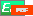, each chapter head is book marked (thus you can jump to the page directly inside PDF) and is also destinated (thus you can jump into the page directly from the present Web page by clicking thebutton shown in the following outline.)PDF file of the Tutorial Lecture:

Tutorial Lecture: USIT (Unified Structured Inventive Thinking) in the Six-Box Scheme

==> PDF file(1.7 MB, 126 pages)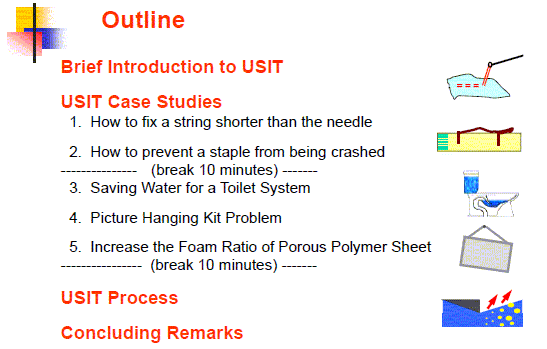Outline of the Tutorial Lecture

Tutorial Lecture: USIT (Unified Structured Inventive Thinking) in the Six-Box SchemeOutline

Brief Introduction to USITUSIT (Unified Structured Inventive Thinking) -- developed by Ed Sickafus
Extension of USIT in Japan ( in 4 steps)
Nakagawa's Recommendations: (Positioning USIT and TRIZ)
Basic scheme for Problem Solving (Conventional: "Four-Box Scheme)
Overall View of the USIT Process ('Six-Box Scheme') (data flow representation)
Overall View of USIT process ("Six box method") (explanation)
USIT Manual （Toru Nakagawa, June 2015）
USIT Case Studies (A Collection of USIT Case Studies: (1) - (5))

USIT Case Study 1. How to fix a string shorter than the needle at the end of sewingReferences of USIT Case Study 1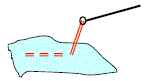Step 1. Define the Problem (1) Preparation: Thesis work
(2) Clarify the problem situations (using the standard template in the USIT Manual)

Step 2: Analyze the Problem (A) Understand the present system
(A1) Understand the Space Characteristics, (A2) Understand the Time Characteristics
Basic Concepts in USIT (Objects, Attributes, Functions)
(A3) Understand the Attributes (properties)
(A4) Understand the Functional Relationships
(A5) Survey for known methods and techniques
Step 2: Analyze the Problem (B) Make an image of the ideal system

Step 3: Generate ideas  (1) By use of the similarity between the known method and the ideal way.
(2) Ideas with breaking the constraints (of properties)

Step 4: Construct solutions:  (a) From the idea of holding the string in the air
(b) From the idea of a pointless needle specialized for making a knot:
(3) Report the results:  Report as a Case Study. Conclusion of the Case Study.

Overview: Whole USIT Process is well illustrated for a familiar problem

USIT Case Study 2. How to prevent a staple from being crashedReferences of USIT Case Study 2.Step 1. Define the Problem (2) Clarify the problem situations

Step 2: Analyze the Problem (A) Understand the present system
(A1) Understand the Space Characteristics, (A2) Understand the Time Characteristics
(A3) Understand the Attributes (properties)
(A4) Understand the Functional Relationships
(A5) Unexpected finding in experiments: The staple was caught in the stapler and ...
The staple was found in an M-shape.  Why M-shape?? --> real root cause
Step 2: Analyze the Problem (B) Make an image of the ideal system:  Should support the staple from inside

Step 3: Generate ideas (1) under the guide of the ideal image: with the SLP method

Step 4: Construct solutions: (2) Construct the conceptual solutions
(3) Report the results:  Report as a Case Study. Conclusion of the Case Study

Overview: A familiar problem was solved by finding the real root cause and by using the SLP method

USIT Case Study 3.  Saving Water for a Toilet SystemReferences of USIT Case Study 3Step 1. Define the Problem (1) Preparation: Development Project
(2) Clarify the problem situations (using the standard template in the USIT Manual)

Step 2: Analyze the Problem (A) Understand the present system
(A1) Understand the Space Characteristics, (A2) Understand the Time Characteristics
(A3) Understand the Attributes (properties), (A4) Understand the Functional Relationships
(A5) Understand the essence of the problem in terms of Contradiction (Physical Contradiction)
Step 2: Analyze the Problem (B) Make an image of the ideal system
(B1) Consider with Separation Principle (Altshuller's method),
(B2) Consider with Particles method (Sickafus' method)
(d) Desirable behaviors (to be performed by the magical Particles), (e) Desirable properties

Step 3: Generate Ideas (1) Generate ideas with the Separation Principle of Physical Contradiction
(2) Basic ideas integrating various ideas stimulated by the problem analysis

Step 4: Construct Solutions (2) Construct the conceptual solutions
(3) Report the results:  Report the results as a development project

Step 5: Implement the Solutions : (Real activities in the 'Real World') Make a prototype of the solution
Performance test and reliability test
Filing a patent and trials of commercialization

Overview:  A familiar, important problem was solved nicely with the concept of Physical Contradiction in TRIZ.

USIT Case Study 4. Picture Hanging Kit ProblemReferences of USIT Case Study 4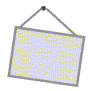Step 1. Define the Problem (2) Clarify the problem situations (using the USIT standard template)

Step 2: Analyze the Problem (A) Understand the present system
(A1) Understand the Space Characteristics
(A2) Understand the Time Characteristics
(A3) Understand the Attributes (properties)
(A4) Understand the Functional Relationships:  A conventional but not-quite right representation
USIT way of functional analysis diagram
Step 2: Analyze the Problem (B) Make an image of the ideal system
(B1) Consider the Ideal system with Separation Principle (Altshuller's method)
(B2) Consider the Ideal system with the Particles Method (Sickafus's method)
(d) Desirable behaviors, (e) Desirable Properties

Step 3: Generate Ideas (1) Write down freely the ideas stimulated by the analyses
Generate various ideas as much as possible, Build them into a hierarchical system
(2) Apply various USIT Operators intentｌｙ
An idea can be interpreted in multiple ways of applying USIT Operators:
(3) Ideas generated by the recognition of the Physical Contradiction

Step 4: Construct Solutions: (1) Evaluate and select ideas, (2) Construct the conceptual solutions
(3) Report the results: Finalize as a Case Study and Report it. Conclusion as the Case Study

Step 5: Implement the Solutions : (Real activities in the 'Real World')

Overview: A standard USIT Case Study on a familiar problem, easy to understand for everybody and yet deep in thoughts

USIT Case Study 5. Increase the Foam Ratio of Porous Polymer SheetReferences of USIT Case Study 5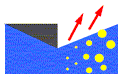Step 1. Define the Problem (1) Preparation: R&D Project in the Real World
USIT Training by use of real problems
(2) Clarify the problem situations and focus the scope
(d) Plausible root causes, (e) Minimal set of relevant objects

Step 2: Analyze the Problem (A) Understand the present system
(A1) Understand the Space Characteristics
(A2) Understand the Time Characteristics
(A3) Understand the Attributes (properties)
(A4) Understand the Functional Relationships
Step 2: Analyze the Problem (B) Make an image of the ideal system
(B2) Consider the Ideal system with the Particles Method (Sickafus' method)
(a) Sketch the present system, (b) Sketch the ideal system (as the result of achievement )
(c) Draw x marks (i.e., Particles) at the places of differences between (a) and (b).
(e) List up Desirable Properties for the Particles may have

Step 3: Generate ideas: (1) Write down the ideas freely with the stimulation of the analyses

Step 4: Construct Solutions (2) Construct the conceptual solutions
(3) Report the results: Finalize as a Case Study and Report it. Conclusion as the Case Study.

Step 5: Implement the Solutions : (Real activities in the 'Real World') Examine and evaluate the results in the parent project.

Overview:  A real problem in the field of chemical engineering is solved with the Particles Method

Overall View of the USIT Process ('Six-Box Scheme') (data flow representation)

Step 1: Define the Problem: (1) Preparation: Form the USIT project
(2) Clarify the problem situations and focus the scope

Step 2: Analyze the Problem (A) Understand the present system
(A1, A2) Understand the space and time characteristics
(A3) Understand the attributes: (Attributes increasing/decreasing (or irrelevant to) the unwanted effect)
(A4) Understand the functional relationships (and understand the designers' intentions and the problems of the present system)
Step 2: Analyze the Problem (B) Make an image of the ideal system (and clarify the directions of solutions)
(B2) Particles Method. (Consider desirable behaviors and desirable properties systematically, with the help of magical Particles)

Step 3: Generate ideas: (1) Write down the ideas stimulated by the analyses (and write them down and build them into a hierarchical diagram.)
"USIT Operators": A system of solution generation methods -- Obtained by re-organizing all the solution methods in TRIZ
System of USIT Operators (5 Main Operators and 32 Sub-Operators)
An example of USIT Operator sub-method (1c) Divide the object (into 1/2, 1/3, ..., 1/Inf.).
(4)  'Solution Combination Method' in USIT
(5) Solution Generalization Method in USIT
How to learn/apply USIT Solution Generation Methods
(2) Extend ideas with USIT operators (Apply various USIT Operators intently to generate more ideas and extend/improve them further)

Step 4: Construct solutions: (1) Evaluate and select ideas (Evaluate a lot of ideas generated so far and select significant ones to be examined and extended further)
(2) Construct the conceptual solutions (On the basis of the capability in the subject matter, construct the conceptual (or preliminary) solutions)
(3) Report the results of the USIT project (Prepare reports of the results of the USIT project, and state proposals.)

Step 5: Implement the solutions: (Real activities in the 'Real World') (Utilize the results of the problem solving project, and implement the new solutions through the activities in the 'Real World')

USIT Case Study 4 (Overview) : Picture Hanging Kit Problem

Concluding RemarksConclusion: ('Six-Box Scheme' is A New Paradigm of Creative Problem Solving.  USIT is a practical procedure for performing the Six-Box Scheme.)
2-Day USIT Training Seminar (Nakagawa)
How to Apply and Practice USIT in Industries
 Top of this page Tutorial Lecture (PDF)Outline Japanese pageGeneral Index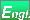(A) Editorial (B) References Links News & activities Software tools (C) Papers, case studies, articles, Lectures, course materials (D) Forum General IndexHome PageNew Informationfor children and highschool students for students and the general public for engineers (introduction) for Practitioners Publications: "TRIZ Practices and Benefits" Series Search in this site Home Page